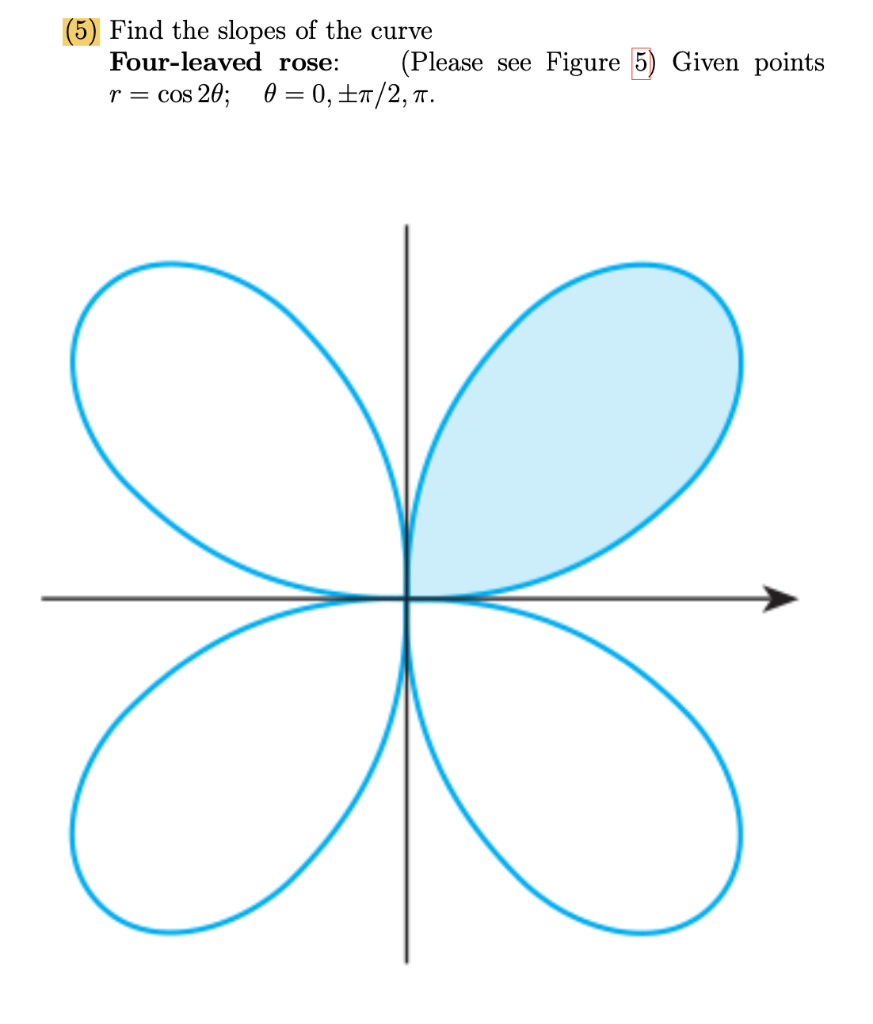### Create an Account

Home / Questions / (5) Find the slopes of the curve Four-leaved rose: (Please see Figure 5) Given points r = ...

# (5) Find the slopes of the curve Four-leaved rose: (Please see Figure 5) Given points r = cos 20; 0 = 0, E7/2,7. B

(5) Find the slopes of the curve Four-leaved rose: (Please see Figure 5) Given points r = cos 20; 0 = 0, E7/2,7. BApr 28 2021 View more View LessSubscribe To Get Solution